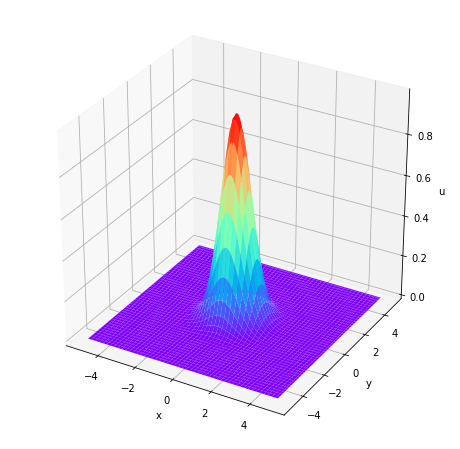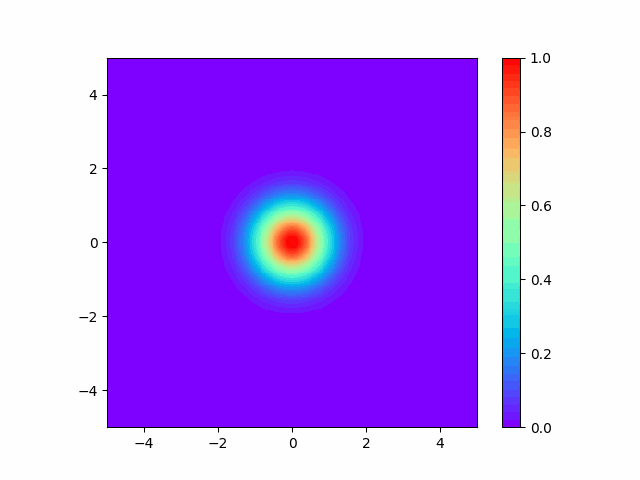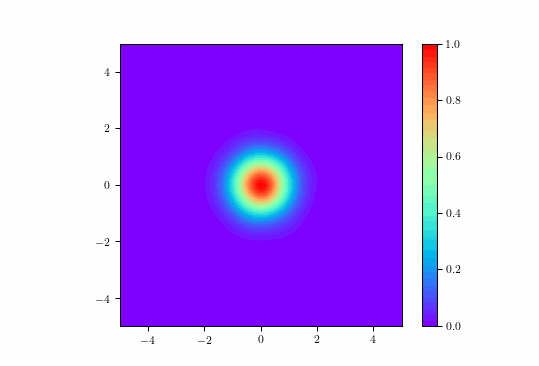# Environment and environment construction

Python == 3.6.8 TensorFlow == 1.15.3

This time, I developed the code using version 1 (TF1) of TensorFlow. TF1 only works with Python 3.6, so I installed Python 3.6.8 first. Install Numpy, Scipy, matplotlib, pyDOE from PyPI (pip command).

# Prerequisites

This time we will solve the diffusion equation. The diffusion equation is u_t - (u_{xx} + u_{yy})=0 The following Gaussian is adopted as the initial condition. u(x,y,0)=exp(-(x^2+y^2))

Gaussian has the following local solution.

``````#Data generation-----------------------------------
xi = np.linspace(-5, 5, 100)
yi = np.linspace(-5, 5, 100)
X, Y = np.meshgrid(xi, yi)
ui = np.exp(-(X**2 + Y**2))

tti = np.zeros(10000)[:,None]
xxi = X.flatten()[:,None]
yyi = Y.flatten()[:,None]
uui = ui.flatten()[:,None]

x_initial = np.hstack([tti, xxi, yyi])
u_initial = uui

#plotting style-----------------------------------
fig = plt.figure(figsize = (8, 8))
ax = fig.add_subplot(111, projection="3d")
ax.plot_surface(X, Y, ui, cmap = "rainbow")
ax.set_xlabel("x")
ax.set_ylabel("y")
ax.set_zlabel("u")
plt.show()
``````It suffices if a solution that spreads this is obtained.

# Source code

``````import tensorflow as tf
import numpy as np
import matplotlib.pyplot as plt
import scipy.io
from scipy.interpolate import griddata
from pyDOE import lhs
from mpl_toolkits.mplot3d import Axes3D
import time
import matplotlib.gridspec as gridspec
from mpl_toolkits.axes_grid1 import make_axes_locatable
import matplotlib.animation as animation

#Fixed randomly generated value---------------------------------
np.random.seed(1234)
tf.set_random_seed(1234)

class PhysicsInformedNN:

#Function to be executed when an instance is created
def __init__(self, X_u, u, X_f, layers, lb, ub):
self.lb = lb
self.ub = ub
self.t_u = X_u[:,0:1]
self.x_u = X_u[:,1:2]
self.y_u = X_u[:,2:3]
self.t_f = X_f[:,0:1]
self.x_f = X_f[:,1:2]
self.y_f = X_f[:,2:3]
self.u = u
self.layers = layers
self.weights, self.biases = self.initialize_NN(layers)
self.sess = tf.Session(config=tf.ConfigProto(allow_soft_placement=True, log_device_placement=True))

self.t_u_tf = tf.placeholder(tf.float32, shape=[None, self.t_u.shape])
self.x_u_tf = tf.placeholder(tf.float32, shape=[None, self.x_u.shape])
self.y_u_tf = tf.placeholder(tf.float32, shape=[None, self.y_u.shape])
self.u_tf = tf.placeholder(tf.float32, shape=[None, self.u.shape])
self.t_f_tf = tf.placeholder(tf.float32, shape=[None, self.t_f.shape])
self.x_f_tf = tf.placeholder(tf.float32, shape=[None, self.x_f.shape])
self.y_f_tf = tf.placeholder(tf.float32, shape=[None, self.y_f.shape])
self.u_pred = self.net_u(self.t_u_tf, self.x_u_tf, self.y_u_tf)
self.f_pred = self.net_f(self.t_f_tf, self.x_f_tf, self.y_f_tf)

self.loss = tf.reduce_mean(tf.square(self.u_tf - self.u_pred)) + \
tf.reduce_mean(tf.square(self.f_pred))
self.optimizer = tf.contrib.opt.ScipyOptimizerInterface(self.loss, method = 'L-BFGS-B',
options = {'maxiter': 50000,
'maxfun': 50000,
'maxcor': 50,
'maxls': 50,
'ftol' : 1.0 * np.finfo(float).eps})
init = tf.global_variables_initializer()
self.sess.run(init)

#Initial weight and bias generation
def initialize_NN(self, layers):
weights = []
biases = []
num_layers = len(layers)
for l in range(0, num_layers-1):
W = self.xavier_init(size=[layers[l], layers[l+1]])
b = tf.Variable(tf.zeros([1,layers[l+1]], dtype=tf.float32), dtype=tf.float32)
weights.append(W)
biases.append(b)
return weights, biases

#Generates a random initial value of weight that follows a truncated normal distribution
def xavier_init(self, size):
in_dim = size
out_dim = size
xavier_stddev = np.sqrt(2/(in_dim + out_dim))
return tf.Variable(tf.truncated_normal([in_dim, out_dim], stddev=xavier_stddev), dtype=tf.float32)

#Building a neural network
def neural_net(self, X, weights, biases):
num_layers = len(weights) + 1
H = 2.0*(X - self.lb)/(self.ub - self.lb) - 1.0
for l in range(0, num_layers-2):
W = weights[l]
b = biases[l]
H = tf.tanh(tf.add(tf.matmul(H, W), b))
W = weights[-1]
b = biases[-1]
return tf.add(tf.matmul(H, W), b)

#Neural network of output u
def net_u(self, t, x, y):
return self.neural_net(tf.concat([t,x, y],1), self.weights, self.biases)

#Neural network of output f(Automatic differentiation)
def net_f(self, t, x, y):
u = self.net_u(t, x, y)
u_t = tf.gradients(u, t)
u_x = tf.gradients(u, x)
u_xx = tf.gradients(u_x, x)
u_y = tf.gradients(u, y)
u_yy = tf.gradients(u_y, y)
#>>>>>>>>The method you want to solve::::::::::::::::::::::::::::::::
return u_t - u_xx - u_yy

#Callback function definition
def callback(self, loss):
print('Loss:', loss)

#Neural network training
def train(self):
tf_dict = {self.x_u_tf: self.x_u,
self.t_u_tf: self.t_u,
self.y_u_tf: self.y_u,
self.u_tf: self.u,
self.x_f_tf: self.x_f,
self.t_f_tf: self.t_f,
self.y_f_tf: self.y_f}
self.optimizer.minimize(self.sess, feed_dict = tf_dict,
fetches = [self.loss], loss_callback = self.callback)

#Output u grid points(X_star)Assign the value of to a variable
def predict(self, X_star):
return self.sess.run(self.u_pred, {self.t_u_tf: X_star[:,0:1],
self.x_u_tf: X_star[:,1:2],
self.y_u_tf: X_star[:,2:3]})

if __name__ == "__main__":

#>>>>>>>Configuration--------------------------------------------------
N_u = 1000   #Number of training data for initial conditions and boundary conditions
N_f = 30000  #Number of collocation points
x0 = -5      #Starting point of x
x1 = 5       #End of x
y0 = -5      #Starting point of y
y1 = 5       #End of y
t0 = 0       #Starting point of t
t1 = 1       #End point of t
layers = [3, 20, 20, 20, 20, 20, 20, 20, 20, 1] #Structure of NN
N = 200      #x,Number of divisions of y
Nt = 100     #Number of divisions of t
#>>>>>>>Configuration--------------------------------------------------

#Initial conditions------------------------------------------------------
xi = np.linspace(x0, x1, N)
yi = np.linspace(y0, y1, N)
Xi, Yi = np.meshgrid(xi, yi)
ui = np.exp(-(Xi**2 + Yi**2))

tti = np.zeros(N*N)[:,None]
xxi = Xi.flatten()[:,None]
yyi = Yi.flatten()[:,None]
uui = ui.flatten()[:,None]

X_u_train = np.hstack([tti, xxi, yyi])
u_train = uui

#Extract as many initial condition data as Nu----------------------------------
idx = np.random.choice(X_u_train.shape, N_u, replace=False)
X_u_train = X_u_train[idx, :]
u_train = u_train[idx,:]

#Generation of 3D coordinate grid points---------------------------------------------
x = np.linspace(x0, x1, N)
y = np.linspace(y0, y1, N)
t = np.linspace(t0, t1, Nt)
X, Y, T = np.meshgrid(x, y, t)

tt = T.flatten()[:,None]
xx = X.flatten()[:,None]
yy = Y.flatten()[:,None]
X_star = np.hstack([tt, xx, yy])

#Generation of collocation points----------------------------------------
lb = np.array([t0, x0, y0])  #<lower bound>
ub = np.array([t1, x1, y1])  #<upper bound>
X_f_train = lb + (ub-lb)*lhs(3, N_f)
X_f_train = np.vstack((X_f_train, X_u_train))

#Pass data to the PhysicsInformedNN class------------------------------
model = PhysicsInformedNN(X_u_train, u_train, X_f_train, layers, lb, ub)

#Execution of operation-------------------------------------------------------
start_time = time.time()
model.train()
run_time = time.time() - start_time
print('Training time:', run_time)

#Store the operation result in a variable--------------------------------------------
u_pred = model.predict(X_star)

#Plotting style-----------------------------------------------
ims = []    #gif animation storage box
fig = plt.figure()
for j in range(Nt):
U = []
for i in range(N*N):
U = np.append(U, u_pred[Nt*i + j])
U = U.reshape(N, N)
im = plt.imshow(U,interpolation='nearest', extent=[x0, x1, y0, y1],
cmap='rainbow', vmin=0, vmax=1)
ims.append([im])
ani = animation.ArtistAnimation(fig, ims, interval=50)
plt.colorbar()
ani.save("output.gif", writer="imagemagick")
plt.show()
``````

# Execution result

The following gif animation was obtained.# Execution result in another equation

The advection equation was solved as another equation. Equation: \$ u_t --u_x -u_y = 0 \$ Initial condition: \$ u (x, y, 0) = exp (-(x ^ 2 + y ^ 2)) \$# reference

I referred to the following papers. Physics Informed Deep Learning (Part I): Data-driven Solutions of Nonlinear Partial Differential Equations